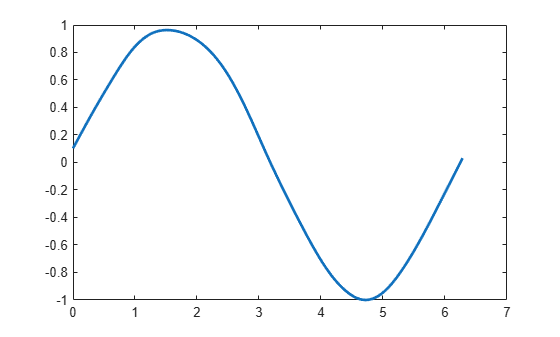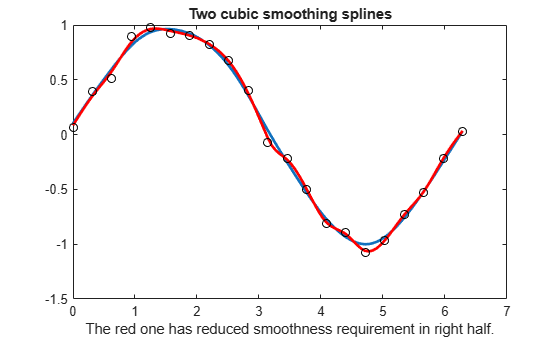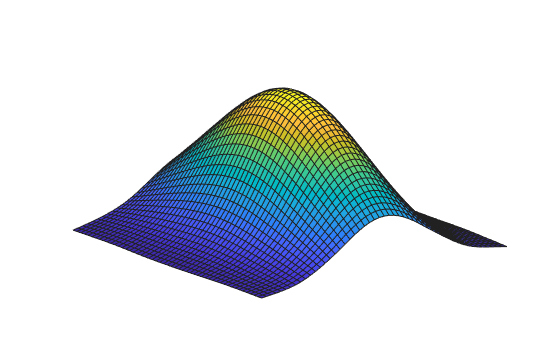# spaps

Smoothing spline

## Syntax

``sp = spaps(x,y,tol) ``
``[sp,values] = spaps(x,y,tol) ``
``[sp,values,rho] = spaps(x,y,tol) ``
``[...] = spaps(x,y,tol,w,m) ``
``[...] = spaps({x1,...,xr},y,tol,...) ``

## Description

example

``` NoteFor a simpler but less flexible method to generate smoothing splines, try the Curve Fitter app or the `fit` function. `sp = spaps(x,y,tol) ` returns the B-form of the smoothest function f that lies within the given tolerance `tol` of the given data points `(x(j), y(:,j)), j=1:length(x)`. The data values `y(:,j)` are scalars, vectors, matrices, or even ND-arrays. Data points with the same data site are replaced by their weighted average, with its weight the sum of the corresponding weights, and the tolerance `tol` is reduced accordingly.```
````[sp,values] = spaps(x,y,tol) ` also returns the smoothed values. `values` is the same as `fnval(sp,x)`.Here, the distance of the function f from the given data is measured by$E\left(f\right)=\sum _{j=1}^{n}w\left(j\right)|\left(y\left(:,j\right)-f\left(x\left(j\right)\right)\right){|}^{2}$with the default choice for the weights `w` making E(f) the composite trapezoidal rule approximation to ${\int }_{\mathrm{min}\left(x\right)}^{\mathrm{max}\left(x\right)}|y-f{|}^{2}$, and |z|2 denoting the sum of squares of the entries of z. Further, smoothest means that the following roughness measure is minimized:$F\left({D}^{m}f\right)=\underset{\mathrm{min}\left(x\right)}{\overset{\mathrm{max}\left(x\right)}{\int }}\lambda \left(t\right)|{D}^{m}f\left(t\right)|{}^{2}dt$where Dmf denotes the `m`th derivative of f. The default value for `m` is `2`, the default value for the roughness measure weight λ is the constant 1, and this makes f a cubic smoothing spline.When `tol` is nonnegative, then the spline f is determined as the unique minimizer of the expression ρE(f) + F(Dmf), with the smoothing parameter ρ (optionally returned) so chosen that E(f) equals `tol`. Hence, when `m` is `2`, then, after conversion to ppform, the result should be the same (up to round-off) as obtained by csaps(x,y,ρ/(ρ + 1)). Further, when `tol` is zero, then the “natural” or variational spline interpolant of order 2m is returned. For large enough `tol`, the least-squares approximation to the data by polynomials of degree <`m` is returned.When `tol` is negative, then ρ is `-tol`.The default value for the weight function λ in the roughness measure is the constant function 1. But you can choose it to be, more generally, a piecewise constant function, with breaks only at the data sites. Assuming the vector `x` to be strictly increasing, you specify such a piecewise constant λ by inputting `tol` as a vector of the same size as `x`. In that case, `tol(i)` is taken as the constant value of λ on the interval (`x(i-1)` .. `x(i)`), `i=2:length(x)`, while `tol(1)` continues to be used as the specified tolerance.```
````[sp,values,rho] = spaps(x,y,tol) ` also returns the actual value of ρ used as the third output argument.```
````[...] = spaps(x,y,tol,w,m) ` lets you specify the weight vector `w` and/or the integer `m`, by supplying them as an `argi`. For this, `w` must be a nonnegative vector of the same size as `x`; `m` must be `1` (for a piecewise linear smoothing spline), or `2` (for the default cubic smoothing spline), or `3` (for a quintic smoothing spline).If the resulting smoothing spline, sp, is to be evaluated outside its basic interval, it should be replaced by `fnxtr(sp,m)` to ensure that its `m`-th derivative is zero outside that interval. ```

example

````[...] = spaps({x1,...,xr},y,tol,...) ` returns the B-form of an `r`-variate tensor-product smoothing spline that is roughly within the specified tolerance to the given gridded data. For scattered data, use `tpaps`. Now `y` is expected to supply the corresponding gridded values, with `size(y)` equal to `[length(x1),...,length(xr)] `in case the function is scalar-valued, and equal to `[d,length(x1),...,length(xr)]` in case the function is `d`-valued. Further, `tol` must be a cell array with `r` entries, with `tol{i}` the tolerance used during the `i`-th step when a univariate (but vector-valued) smoothing spline in the `i`-th variable is being constructed. The optional input for `m `must be an `r`-vector (with entries from the set `{1,2,3}`), and the optional input for `w `must be a cell array of length `r`, with `w{i}` either empty (to indicate that the default choice is wanted) or else a positive vector of the same length as `xi`.```

## Examples

collapse all

This code returns a fit to the noisy data that is expected to be quite close to the underlying noisefree data since the latter come from a slowly varying function and since the used `TOL` is of the size appropriate for the size of the noise.

```x = linspace(0,2*pi,21); y = sin(x) + (rand(1,21)-.5)*.2; sp = spaps(x,y, (.05)^2*(x(end)-x(1)) ); fnplt(sp)```This code uses the same data and tolerance as before, but chooses the roughness weight to be only `0.1` in the right half of the interval and gives, correspondingly, a rougher but better fit there.

```sp1 = spaps(x,y, [(.025)^2*(x(end)-x(1)),ones(1,10),repmat(.1,1,10)] ); fnplt(sp1)```Finally, compare the two cubic smoothing splines obtained previously.

```fnplt(sp); hold on fnplt(sp1,'r') plot(x,y,'ok') hold off title('Two cubic smoothing splines') xlabel('The red one has reduced smoothness requirement in right half.')```This example produces a smooth approximant to noisy data from a smooth bivariate function. Note the use of `ndgrid` here; use of `meshgrid` would produce an error.

```x = -2:.2:2; y=-1:.25:1; [xx,yy] = ndgrid(x,y); rng(39); z = exp(-(xx.^2+yy.^2)) + (rand(size(xx))-.5)/30; sp = spaps({x,y},z,8/(60^2)); fnplt(sp) axis off```## Input Arguments

collapse all

Data sites of data values `y` to be fit, specified as a vector or as a cell array for multivariate data. Spline f is created with knots at each data site `x` such that f(`x(j)`) = `y(:,j)` for all values of `j`.

For multivariate, gridded data, you can specify `x` as a cell array that specifies the data site in each variable dimension: f(`x1(i),x2(j),...xn(k)`) = `y(:,i,j,...,k)`.

Data Types: `single` | `double`

Data values to fit during creation of the spline, specified as a vector, matrix, or array. Data values `y(:,j)` can be scalars, matrices, or n-dimensional arrays. Data values given at the same data site `x` are averaged.

Data Types: `single` | `double`

Tolerance of the given data points, specified as a scalar.

Data Types: `single` | `double`

Weights w of the data points, specified as a vector of nonnegative entries of the same size as `x`.

Derivative order, specified as a scalar. This value must be either `1` for a piecewise linear smoothing spline, `2` for the default cubic smoothing spline, or `3` for a quintic smoothing spline.

Data Types: `single` | `double`

## Output Arguments

collapse all

Spline in B-form, returned as a structure with these fields.

Form of the spline, returned as `B-`. `B-` indicates that the spline is given in B-form.

Knot positions of the spline, returned as a vector or as a cell array of vectors for multivariate data. Vectors contain strictly increasing elements that represent the start and end of each of the intervals over which the polynomial pieces are defined.

Coefficients of polynomials for each piece, returned as a matrix or as an array for multivariate data.

Number of polynomial pieces describing the spline, returned as a scalar or as a vector of numbers of pieces in each variable for multivariate data.

Order of the polynomial function describing each polynomial piece of the spline, returned as a scalar or as a vector containing the order in each variable for multivariate data.

Dimensionality of the target function, returned as a scalar.

Values of the spline at the points in `x`, returned as a vector or as a matrix or array for multivariate data.

Smoothing parameter used to calculate the spline, returned as a scalar or as a cell array of scalar values for multivariate data.

## Algorithms

This function uses the Reinsch's approach  , including his way of choosing the equation for the optimal smoothing parameter in such a way that a good initial guess is available and Newton's method is guaranteed to converge and to converge fast.

 C. Reinsch. "Smoothing by spline functions." Numer. Math. 10 (1967), 177–183.